# SQL Server中的“+”号的用法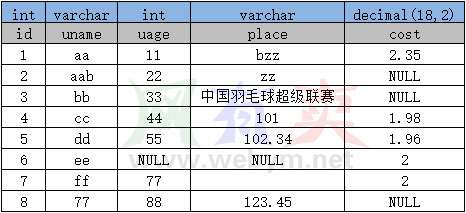### 数字 + 字符串

int + varchar

```SELECT id + place FROM Users WHERE id = 1;  //提示错误“在将 varchar 值 'bzz' 转换成数据类型 int 时失败”
SELECT id + place FROM Users WHERE id = 5;  //提示错误“在将 varchar 值 '102.34' 转换成数据类型 int 时失败”
SELECT id + place FROM Users WHERE id = 4;	//返回int “105”
```

decimal + varchar

```SELECT *, place + cost  FROM Users WHERE id = 4 OR id = 5;	//返回decimal “102.98”和“104.30”
SELECT *, place + cost FROM Users WHERE id = 1;	//提示错误“从数据类型 varchar 转换为 numeric 时出错。”
```

### 数字 + 数字

```SELECT *,  uage + cost AS 'uage + cost'  FROM Users
```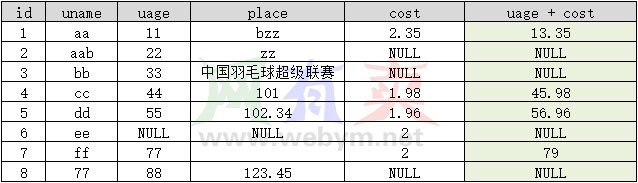### 字符串 + 字符串

```SELECT *, uname + place AS 'uname + place' FROM Users
```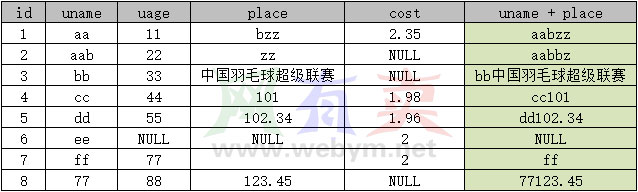### 使用CAST和CONVERT函数进行类型转换

• CAST()函数可以将某种数据类型的表达式转化为另一种数据类型
• CONVERT()函数也可以将制定的数据类型转换为另一种数据类型

```SELECT CAST('678' AS INT) + 123
SELECT CONVERT(INT, '678') + 123
```

```SELECT *, CONVERT(varchar(10), id) + place FROM Users
```

```SELECT * FROM Users WHERE uname + place = 'aabzz'
```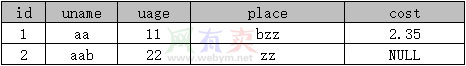```SELECT * FROM Users WHERE uname = 'aa' AND place = 'bzz'
```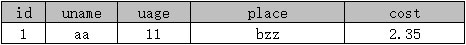```SELECT * FROM Users WHERE uname + 'rain@&%\$man' + place = 'aa' + 'rain@&%\$man' + 'bzz'
```
• 暂无评论